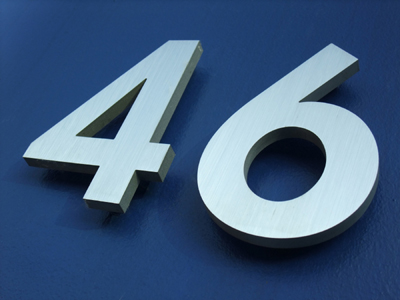If 6 - 5 = 1, then 46 - 5 = 41.

# Adding and Subtracting One-Digit Numbers from Two-Digit Numbers

This Math quiz is called 'Adding and Subtracting One-Digit Numbers from Two-Digit Numbers' and it has been written by teachers to help you if you are studying the subject at elementary school. Playing educational quizzes is a fun way to learn if you are in the 1st or 2nd grade - aged 6 to 8.

It costs only \$12.50 per month to play this quiz and over 3,500 others that help you with your school work. You can subscribe on the page at Join Us

Adding and subtracting one digit numbers from a two digit number follows on closely from the skill of adding and subtracting using only one digit numbers. At first, this calculating may rely on using equipment or fingers to check an answer, but will gradually progress to using only a number line and then perhaps visualizing that line mentally.

Try this quiz to see how well you add or subtract.

1.
What is 51 + 8?
59
60
68
54
Changing the units in the calculation to 58 + 1 might make it easier, and still gives the same answer
2.
What is 20 - 8?
2
18
22
12
Knowing number bonds to 20 is useful for subtractions such as this
3.
What is 60 - 8?
62
58
52
68
Knowing number bonds well is useful in all sorts of calculations
4.
What is 46 - 9?
37
56
36
29
Taking away 10 and then adding back a 1 is useful when subtracting 9
5.
What is 5 + 32?
73
325
532
37
Again, changing the numbers round makes the calculation easier, and still gives the same answer
6.
What is 55 - 7?
63
47
48
50
Counting back in two jumps (of 5 and 2) makes this calculation easier
7.
What is 34 - 5?
39
27
29
30
Try to picture this calculation as 34 - 4 - 1 to make it easier to calculate mentally
8.
What is 42 + 8?
52
48
44
50
Knowing number bonds may help to recognize that 2 + 8 = 10
9.
What is 12 - 7?
5
6
4
19
Counting up from 7 to 12 would give the same answer as counting back from 12 to 7
10.
What is 4 + 20?
42
24
204
420
This addition could be done in a different order to make it easier: 20 + 4
Author:  Angela Smith